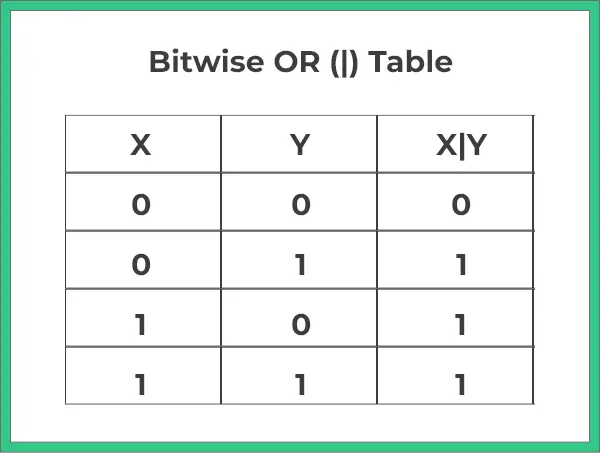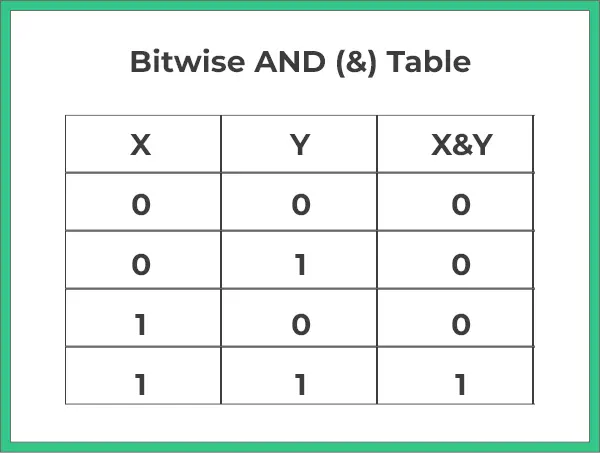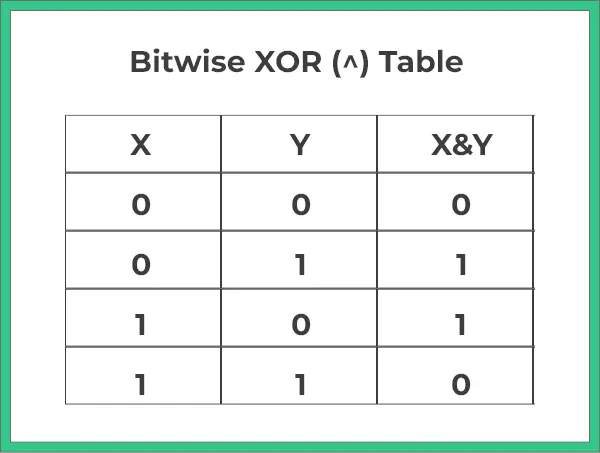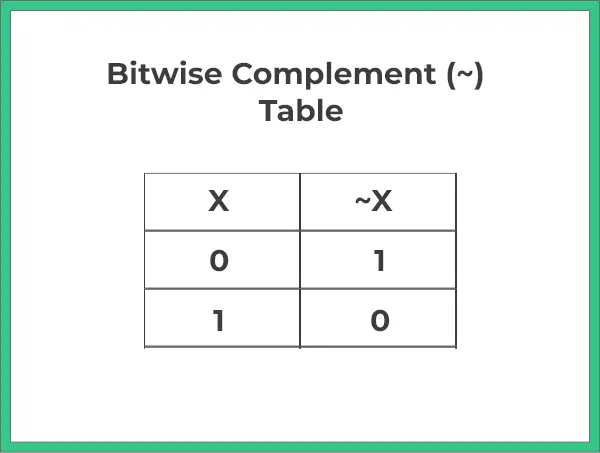# Bitwise Operators In Java## Bitwise Operators

Bitwise Operators in Java form the basic building blocks of all programming languages. Java also provides a wide variety of operators, including logical, arithmetic, and relational, that you can use as needed to perform different calculations and functions.

There are several types of bitwise operators in java that we will be going to be discuss in this Page.

## Types of Bitwise Operators :

 Operator Functionality Syntax Bitwise OR operator The result of OR is 1 if any bit is 1 a | b Bitwise AND operator The result of AND is 1 if both bits are 1 a & b Bitwise XOR operator The result of XOR is 1 if the two bits are different. a ^ b Bitwise Complement Operator It takes one number and inverts all bits of it a ~ b

## Bitwise OR:

Bitwise OR is an operator is a binary operator denoted by (|). Returns the bitwise OR of the input values. That is, it returns 1 if any bit is 1, and 0 otherwise.Run
```import java.util.*;

public class Main{
public static void main(String[] args){
int a = 8;
int b = 9;

int ans = a | b;
System.out.println(ans);
}
}```
```Output :
9```

## Bitwise AND

This operator is a binary operator, denoted by ‘&.’ It returns bit by bit AND of input values, i.e., if both bits are 1, it gives 1, else it shows 0.Run
```import java.util.*;

public class Main{
public static void main(String[] args){
int a = 8;
int b = 9;

int ans = a & b;
System.out.println(ans);
}
}```
```Output :
8```

## Bitwise XOR

This operator is a binary operator, denoted by ‘^.’ It returns bit by bit XOR of input values, i.e., if corresponding bits are different, it gives 1, else it shows 0.Run
```import java.util.*;

public class Main{
public static void main(String[] args){
int a = 8;
int b = 9;

int ans = a ^ b;
System.out.println(ans);
}
}```
```Output :
1```

## Bitwise Complement

This operator is a unary operator, denoted by ‘~.’ It returns the one’s complement representation of the input value, i.e., with all bits inverted, which means it makes every 0 to 1, and every 1 to 0.Run
```import java.util.*;

public class Main{
public static void main(String[] args){
int a = 8;

int ans = ~a;
System.out.println(ans);
}
}```
```Output :
-9```

### Related Banners

Get PrepInsta Prime & get Access to all 200+ courses offered by PrepInsta in One Subscription

## Get over 200+ course One Subscription

Courses like AI/ML, Cloud Computing, Ethical Hacking, C, C++, Java, Python, DSA (All Languages), Competitive Coding (All Languages), TCS, Infosys, Wipro, Amazon, DBMS, SQL and others